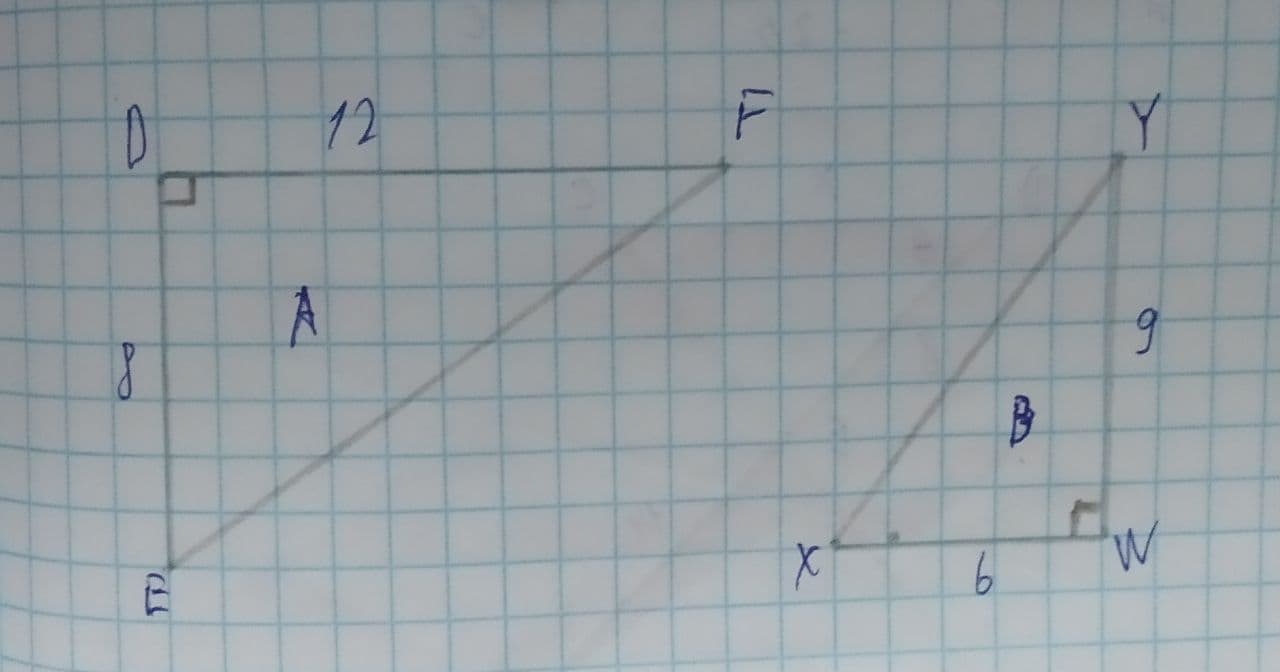# Identify a similarity statement for the two triangles.jernplate8 2021-08-01 Answered
Identify a similarity statement for the two triangles.1.The triangles are not similar
2.$\mathrm{△}DEF\sim \mathrm{△}WXY$
3.$\mathrm{△}FED\sim \mathrm{△}WXY$
4.$\mathrm{△}DEF\sim \mathrm{△}XYW$
5.$\mathrm{△}FED\sim \mathrm{△}XYW$
You can still ask an expert for help

• Questions are typically answered in as fast as 30 minutes

Solve your problem for the price of one coffee

• Math expert for every subject
• Pay only if we can solve itfaldduE
Step 1
Two triangles DEF and WXY is given in the problem.
The ratio of side DE and WX is,
$\frac{DE}{XW}=\frac{8}{6}$
=1.33
The ratio of side FD and YW is,
$\frac{FD}{YW}=\frac{12}{9}$
=1.33
Step 2
The angle between the corresponding sides, are the same right angles.
So,
1) The ratio of the two corresponding sides of the triangle is the same for two pair sides.
2) The angle between the two corresponding sides of the triangles are congruent.
The above statements satisfy the condition of the SAS similarity rule. So, $\mathrm{△}DEF\sim \mathrm{△}WXY$.
Thus, the second option "$\mathrm{△}DEF\sim \mathrm{△}WXY$" is the correct option.